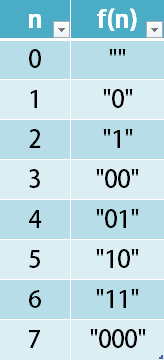## 1256. Encode Number

Given a non-negative integer `num`, Return its encoding string.

The encoding is done by converting the integer to a string using a secret function that you should deduce from the following table:Example 1:

```Input: num = 23
Output: "1000"
```

Example 2:

```Input: num = 107
Output: "101100"
```

Constraints:

• `0 <= num <= 10^9`

## Rust Solution

``````struct Solution;

impl Solution {
fn encode(num: i32) -> String {
format!("{:b}", num + 1)[1..].to_string()
}
}

#[test]
fn test() {
let num = 23;
let res = "1000".to_string();
assert_eq!(Solution::encode(num), res);
let num = 107;
let res = "101100".to_string();
assert_eq!(Solution::encode(num), res);
}
``````

Having problems with this solution? Click here to submit an issue on github.